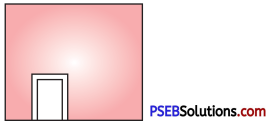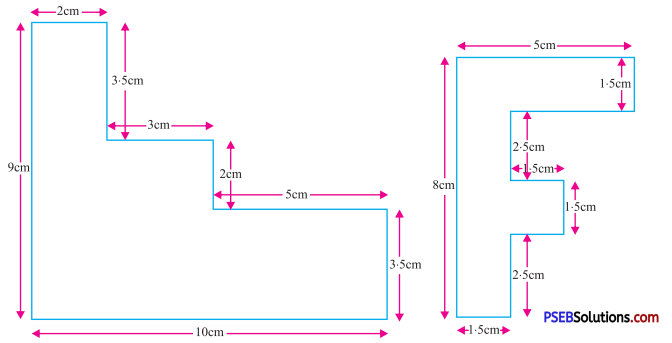# PSEB 7th Class Maths Solutions Chapter 11 Perimeter and Area Ex 11.1

Punjab State Board PSEB 7th Class Maths Book Solutions Chapter 11 Perimeter and Area Ex 11.1 Textbook Exercise Questions and Answers.

## PSEB Solutions for Class 7 Maths Chapter 11 Perimeter and Area Ex 11.1

1. Find the perimeter and the area of a rectangle having :
(i) Length = 28 cm, Breadth = 15 cm
(ii) Length = 9.4 cm Breadth = 2.5 cm
Solution:
(i) Given length of rectangle = 28 cm
Breadth of rectangle = 15 cm
Perimeter of rectangle = 2 [length + Breadth]
= 2 [28 + 15]
= 2 × 43
= 86 cm

Area of rectangle = length × Breadth
= 28 × 15
= 420 cm2

(ii) Perimeter of rectangle = 2 [9.4 + 2.5]
= 2 × 11.9
= 23.8 cm
Area of rectangle = 9.4 × 2.5
= 23.5 cm2

2. Find the perimeter and the area of a square whose side measures
(i) 29 cm
(ii) 8.3 cm
Solution:
(i) Given side of square = 29 cm
Perimeter of square = 4 × side
= 4 × 29
= 116 cm
Area of square = (side)2
= (29)2
= 841 cm2

(ii) Perimeter of square = 4 × 8.3
= 33.2 cm
Area of square = 8.3 × 8.3
= 68.89 cm23. The perimeter of a square park is 148 m. Find its area.
Solution:
Given the perimeter of square park = 148 m
Side of the square park = $$\frac{perimeter}{4}$$
= $$\frac {148}{4}$$
Area of the square park = (side)2
= (37)2
= 1369 m2

4. The area of a rectangle is 580 cm2. Its length is 29 cm. Find its breadth and also, the perimeter.
Solution:
Given area of rectangle = 580 cm2
Length of the rectangle = 29 cm
Let breadth of the rectangle = b cm
Area of the rectangle = length × breadth
580 = 29 × b
$$\frac {580}{29}$$ = b
b = 20 cm
Perimeter of rectangle = 2 [length + breadth]
= 2 [29 + 20]
= 2 × 49
= 98 cm

5. A wire is in the shape of a rectangle. Its length is 48 cm and breadth is 32 cm. If the same wire is rebent into the shape of a square, what will be the measure of each side. Also, find which shape encloses more area and by how much ?
Solution:
Given length of the rectangle = 48 cm
Breadth of the rectangle = 32 cm
Perimeter of the rectangle = 2 [length + breadth]
= 2 [48 + 32]
= 2 × 80
= 160 cm
Let side of square = a cm
Perimeter of the square = 4 × a
Since wire is rebent into the shape of a square
Perimeter of square = Perimeter of rectangle
4 a = 160
Therefore, a = $$\frac {160}{4}$$
= 40 cm
Area of square = (side)2
= 40 × 40
= 1600 cm2
Area of rectangle = length × breadth
= 48 × 32
= 1536 cm2
∴ Square encloses more area by 64 cm26. The area of a square park is the same as that of a rectangular park. If the side of the square park is 75 m and the length of the rectangular park is 125 m, find the breadth of the rectangular park. Also, find the perimeter of rectangular park.
Solution:
Given side of square park = 75 m
Area of square park = (75)2
= 75 × 75
= 5625 m2
Length of rectangular park = 125 m
Let breadth of rectangular park = b m
Area of rectangular park = length × breadth
= 125 × b m2
Given that
Area of rectangular park = Area of square park
125 × b = 5625
= 45 m
Perimeter of rectangular park = 2 [length + breadth]
= 2 [125 + 45]
= 2 × 170
= 340 m

7. A door of length 2.5 m and breadth 1.5 m is fitted in a wall. The length of wall, is 9 m and breadth is 6 m. Find the cost of painting the wall, if the rate of painting the wall is ₹ 30 per m2.Solution:
Length of door = 2.5 m
Breadth of door = 1.5 m
Area of door = length × breadth
= 2.5 × 1.5
= 3.75 m2
Area of wall = 9 × 6
= 54 m2
Area of wall painting = Area of wall including door – Area of door
= 54 – 3.75
= 50.25 m2
Cost of painting 1 m2 of wall = ₹ 30
Cost of painting 50.25 m2 of wall = ₹ 50.25 × 30
= ₹ 1507.508. A door of dimensions 3 m × 2 m and a window of dimensions 2.5 m × 1.5 m is fitted in a wall. The length of the wall is 7.8 m and breadth is 3.9 m. Find the cost of painting the wall, if the rate of painting the wall is ₹ 25 per m2.
Solution:
Area of door = 3 × 2 = 6 m2
Area of window = 2.5 m × 1.5 m
= 3.75 m2
Area of wall = 7.8 m × 3.9 m
= 30.42 m2
Area of painting the wall = Area of wall – Area of door – Area of window
= 30.42 – 6 – 3.75
= 20.67 m2
Cost of painting the wall = ₹ 25 × 20.67
= ₹ 516.75

9. Find the area and the perimeter of the following figures.Solution:
(i) Perimeter of the given figure
= AB + BC + CD + DE + EF + FG + GH + HA
= 2 + 3.5 + 3 + 2 + 5 + 3.5 + 10 + 9
= 38 cm2
Area of the figure = Area of rectangle ABCJ + Area of rectangle JDEI + Area of rectangle IFGH
= 2 × 3.5 + 5 × 2 + 10 × 3.5
= 7 + 10 + 35
= 52 cm2

(ii) Perimeter of the given figure
= 8cm + 5 cm + 1.5 cm + 2.5 cm + 2.5 cm + 1.5 cm + 1.5 cm + 1.5 cm + 2.5 cm + 1.5 cm
= 29 cm
Area of the given figure = Area of rectangle I + Area of rectangle II + Area of rectangle III
= 8 cm × 1.5 cm + 3.5 cm × 1.5 cm + 1.5 cm × 1.5 cm
= 12 cm2 + 5.25 cm2 + 2.25 cm
= 19.5 cm210. Multiple Choice Questions :

Question (i).
What is the area of a rectangle of dimensions 12 cm × 10 cm ?
(a) 44 cm2
(b) 120 cm2
(c) 1200 cm2
(d) 1440 cm2
(b) 120 cm2

Question (ii).
Find the breadth of a rectangle whose length is 12 cm and perimeter is 36 cm.
(a) 6 cm
(b) 3 cm
(c) 9 cm
(d) 12 cm
(a) 6 cm

Question (iii).
If each side of a square is 1 m then its area is ?
(a) 10 cm2
(b) 100 cm2
(c) 1000 cm2
(d) 10000 cm2
(d) 10000 cm2

Question (iv).
Find the area of a square whose perimeter is 96 cm.
(a) 576 cm2
(b) 626 cm2
(c) 726 cm2
(d) 748 cm2.
(a) 576 cm2

Question (v).
The area of a rectangular sheet is 500 cm2. If the length of the sheet is 25 cm, what is its breadth ?
(a) 30 cm
(b) 40 cm
(c) 20 cm
(d) 25 cm.
(b) The area becomes $$\frac {1}{4}$$ times, the area of original square.
(d) The area becomes $$\frac {1}{6}$$ times, 6 the area of original square.